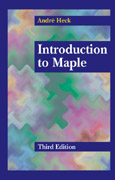# Introduction to Maple, 3rd Edition.### ISBN 0-387-00230-8

844 pp, 221 figs., 52 tables.

## About the Revised and Expanded Edition

The first two editions of this book have been very well received by the community, but so many revisions of the Maple system have occurred since then that simply reprinting the out-of-stock book would not do anymore. A major revision of the book was inevitable, too. The wording "major revision" must be taken seriously because I not only corrected typographical errors, rephrased text fragments, and updated many examples, but I also rewrote complete chapters and added new material. In particular, the chapter on differential equations now discusses Lie symmetry methods, numerical methods, and partial differential equations. Linear algebra is based throughout the book on the packages `LinearAlgebra` and `VectorCalculus`, which replace the deprecated package `linalg`. Maple users are strongly advised to do their work with the new packages. The chapter on simplification has been updated and expanded; it discusses the use of assumptions in more detail now. Many of the Maple sessions have been rewritten so that they comply with the most recent version of Maple, viz., Maple 8. As a result of all this work, hardly any section in the book has been left untouched.

## Contents

Completely new and strongly revised sections are emphasized below.

1. Introduction to Computer Algebra
1. What is Computer Algebra?
2. Computer Algebra Systems
3. Some Properties of Computer Algebra Systems
4. Advantages of Computer Algebra
5. Limitations of Computer Algebra
6. Design of Maple

2. The First Steps: Calculus on Numbers
1. Getting Started
2. Getting Help
3. Integers and Rational Numbers
4. Irrational Numbers and Floating-Point Numbers
5. Algebraic Numbers
6. Complex Numbers
7. Exercises

3. Variables and Names
1. Assignment and Unassignment
2. Evaluation
3. Names of Variables
4. Basic Data Types
5. Attributes
6. Properties
7. Exercises

4. Getting Around with Maple
1. Maple Input and Output
2. The Maple Library
3. Reading and Writing Files
4. Importing and Exporting Numerical Data
5. Low-Level I/O
6. Code Generation
7. Changing Maple to your own Taste
8. Exercises

5. Polynomials and Rational Functions
1. Univariate Polynomials
2. Multivariate Polynomials
3. Rational Functions
4. Conversions
5. Exercises

6. Internal Data Representation and Substitution
1. Internal Representation of Polynomials
2. Generalized Rational Expressions
3. Substitution
4. Exercises

7. Manipulation of Polynomials and Rational Expressions
1. Expansion
2. Factorization
3. Canonical Form and Normal Form
4. Normalization
5. Collection
6. Sorting
7. Exercises

8. Functions
1. Mathematical Functions
2. Arrow Operators
3. Piecewise Defined Functions
4. Maple Procedures
5. Recursive Procedure Definitions
6. `unapply`
7. Operations on Functions
8. Anonymous Functions
9. Exercises

9. Differentiation
1. Symbolic Differentiation
2. Automatic Differentiation
3. Exercises

10. Integration and Summation
1. Indefinite Integration
2. Definite Integration
3. Numerical Integration
4. Integral Transforms
5. Assisting Maple's Integrator
6. Summation
7. Exercises

11. Series, Approximation, and Limits
1. Truncated Series
2. Approximation of Functions
3. Power Series
4. Limits
5. Exercises

12. Composite Data Types
1. Sequence
2. Set
3. List
4. Arrays
5. Table: `table`
6. Last Name Evaluation
7. Rectangular Table: `rtable`
8. Record Data Structure
9. Function Call
10. Conversion between Composite Data Types
11. Exercises

13. The Assume Facility
1. The Need of an Assume Facility
2. Basics of assume
3. An Algebra of Properties
4. Implementation of assume
5. Exercises
6. Hierarchy of Properties

14. Simplification
1. Automatic Simplification
2. `expand`
3. `combine`
4. `simplify`
5. `convert`
6. Trigonometric Simplification
7. Simplification w.r.t. Side Relations
8. Control over Simplification
9. Defining Your Own Simplification Routines
10. Exercises
11. Simplification Chart

15. Graphics
1. Some Basic Two-Dimensional Plots
2. Options of plot
3. The Structure of Two-Dimensional Graphics
4. The `plottools` Package
5. Special Two-Dimensional Plots
6. Two-dimensional Geometry
7. Plot Aliasing
8. A Common Mistake
9. Some Basic Three-Dimensional Plots
10. Options of `plot3d`
11. The Structure of Three-Dimensional Graphics
12. Special Three-Dimensional Plots
13. Data Plotting
14. Animation
15. List of Plot Options
16. Exercises.

16. Solving Equations
1. Equations in One Unknown
2. Abbreviations in solve
3. Some Difficulties
4. Systems of Equations
5. The Gröbner Basis Method
6. Inequalities
7. Numerical Solvers
8. Other Solvers in Maple
9. Exercises

17. Differential Equations
1. First Glance at ODEs
2. Analytic Solutions
3. Lie Point Symmetries for ODEs
4. Taylor Series Method
5. Power Series Method
6. Numerical Solutions
7. Graphical Methods
8. Change of Coordinates
9. Perturbation Methods
10. Partial Differential Equations
11. Lie Point Symmetries of PDEs
12. Exercises

18. The `LinearAlgebra Package`
1. Loading the `LinearAlgebra` Package
2. Creating Vectors and Matrices
3. Vector and Matrix Arithmetic
4. Basic Matrix Functions
5. Structural Operations
6. Vector Operations
7. Standard Forms of Matrices
8. Numeric Linear Algebra
9. Exercises

19. Linear Algebra: Applications
1. Kinematics of the Stanford Manipulator
2. A 3-Compartment Model of Cadmium Transfer
3. Molecular-orbital Hückel Theory
4. Vector Analysis
5. Moore-Penrose Inverse
6. Exercises

20. A Bird's Eye View of Gröbner Bases
1. Introduction
2. Elementary Solution Methods
1. Heuristic Method
2. Gaussian Elimination-Like Method
3. Conclusion
3. Basics of the Gröbner Basis Method
1. Term Ordering
2. Polynomial Reduction and Normal Form
3. Characterization of a Gröbner Basis
4. The Buchberger Algorithm
5. Improvements of Buchberger's Algorithm
4. Properties and Applications of Gröbner Bases
1. Equivalence of Systems of Polynomial Equations
2. Dimension, Hilbert Series and Hilbert Polynomial
3. Solvability of Polynomial Equations
4. Finite Solvability of Polynomial Equations
5. Counting of Finite Solutions
6. Converting a System of Polynomial Equation into Triangular Form
7. Finding a Univariate Polynomial
8. Decomposition of Ideals
9. An Example from Robotics
10. Implicitization of Parametric Objects
11. Invertibility of Polynomial Mappings
12. Simplification of Expressions
13. Working over General Algebras
5. Exercises
• References
• Index